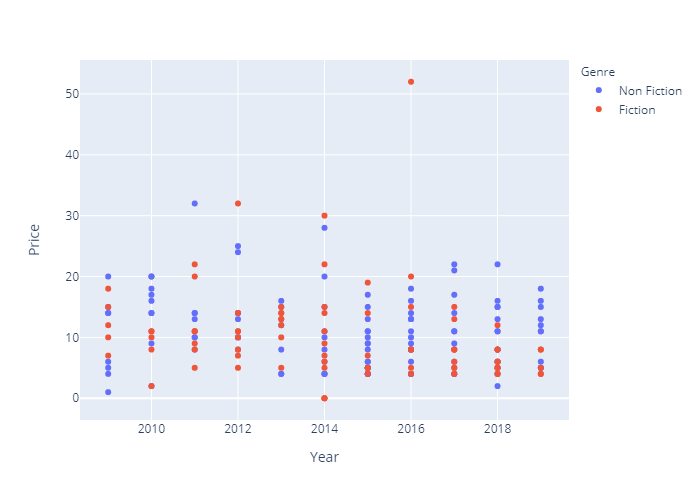# Python Plotly – Exporting to Static Images

• Last Updated : 19 Dec, 2021

In this article, we will discuss how to export plotly graphs as static images using Python.

To get the job done there are certain additional installations that need to be done. Apart from plotly, orca and psutil have to be installed.

• psutil (python system and process utilities) is a cross-platform Python package that retrieves information about running processes and system utilisation.  It can be installed as follows.
`pip install psutil`
• ocra cannot be installed via pip so conda is employed for the same.
`conda install -c plotly plotly-orca psutil`

Once all installations are successful, we have to import plotly.io when importing other required modules and use, write_image() function to save the output plot.

Syntax:

`write_image(figure, path)`

Parameter:

• figure: plot
• format: location to save along with the format

Plotly images can be made static if they are exported either as images or in vector format. The only difference is specifying the format while importing.

Example:

In this example, we export a plotly graph as an image in python.

Dataset used here: bestsellers.csv

## Python3

 `# import libraries ` `import` `plotly.express as px ` `import` `pandas as pd ` `import` `plotly.io as pio ` ` `  `# read dataset ` `data ``=` `pd.read_csv(``"bestsellers.csv"``) ` ` `  `# create plot ` `fig ``=` `px.scatter(data, x``=``"Year"``, y``=``"Price"``, color``=``"Genre"``) ` ` `  `# export as static image ` `pio.write_image(fig, ``"op.png"``) `

Output:Example: In this example, we export plotly graph as a vector image in python.

## Python3

 `# import libraries ` `import` `plotly.express as px ` `import` `pandas as pd ` `import` `plotly.io as pio ` ` `  `# read dataset ` `data ``=` `pd.read_csv(``"bestsellers.csv"``) ` ` `  `# create plot ` `fig ``=` `px.scatter(data, x``=``"Year"``, y``=``"Price"``, color``=``"Genre"``) ` ` `  `# export as static image ` `pio.write_image(fig, ``"op.pdf"``) `

Output:My Personal Notes arrow_drop_up
Recommended Articles
Page :